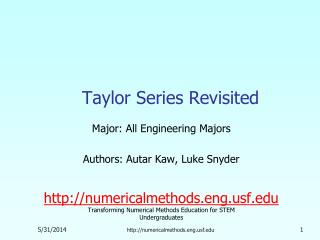DownloadDownload PresentationTaylor Series Revisited

# Taylor Series Revisited

Télécharger la présentation## Taylor Series Revisited

- - - - - - - - - - - - - - - - - - - - - - - - - - - E N D - - - - - - - - - - - - - - - - - - - - - - - - - - -
##### Presentation Transcript

1. Taylor Series Revisited Major: All Engineering Majors Authors: Autar Kaw, Luke Snyder http://numericalmethods.eng.usf.edu Transforming Numerical Methods Education for STEM Undergraduates http://numericalmethods.eng.usf.edu

2. Taylor Series Revisitedhttp://numericalmethods.eng.usf.edu

3. What is a Taylor series? Some examples of Taylor series which you must have seen http://numericalmethods.eng.usf.edu

4. General Taylor Series The general form of the Taylor series is given by provided that all derivatives of f(x) are continuous and exist in the interval [x,x+h] What does this mean in plain English? As Archimedes would have said, “Give me the value of the function at a single point, and the value of all (first, second, and so on) its derivatives at that single point, and I can give you the value of the function at any other point” (fine print excluded) http://numericalmethods.eng.usf.edu

5. Example—Taylor Series Find the value of given that and all other higher order derivatives of at are zero. Solution: http://numericalmethods.eng.usf.edu

6. Example (cont.) Solution: (cont.) Since the higher order derivatives are zero, Note that to find exactly, we only need the value of the function and all its derivatives at some other point, in this case http://numericalmethods.eng.usf.edu

7. Derivation for Maclaurin Series for ex Derive the Maclaurin series The Maclaurin series is simply the Taylor series about the point x=0 http://numericalmethods.eng.usf.edu

8. Derivation (cont.) Since and the Maclaurin series is then So, http://numericalmethods.eng.usf.edu

9. Error in Taylor Series The Taylor polynomial of order n of a function f(x) with (n+1) continuous derivatives in the domain [x,x+h] is given by where the remainder is given by where that is, c is some point in the domain [x,x+h] http://numericalmethods.eng.usf.edu

10. Example—error in Taylor series The Taylor series for at point is given by It can be seen that as the number of terms used increases, the error bound decreases and hence a better estimate of the function can be found. How many terms would it require to get an approximation of e1 within a magnitude of true error of less than 10-6. http://numericalmethods.eng.usf.edu

11. Example—(cont.) Solution: Using terms of Taylor series gives error bound of Since http://numericalmethods.eng.usf.edu

12. Example—(cont.) Solution: (cont.) So if we want to find out how many terms it would require to get an approximation of within a magnitude of true error of less than , So 9 terms or more are needed to get a true error less than http://numericalmethods.eng.usf.edu

13. Additional Resources For all resources on this topic such as digital audiovisual lectures, primers, textbook chapters, multiple-choice tests, worksheets in MATLAB, MATHEMATICA, MathCad and MAPLE, blogs, related physical problems, please visit http://numericalmethods.eng.usf.edu/topics/taylor_series.html

14. THE END http://numericalmethods.eng.usf.edu